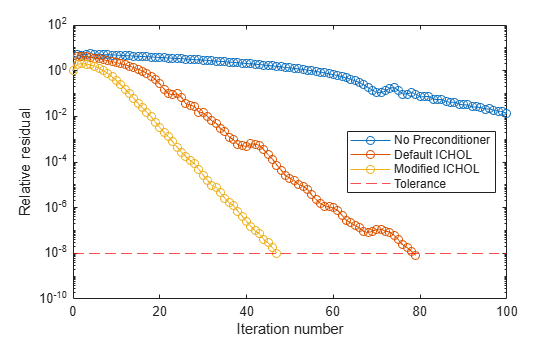Documentation

# pcg

Preconditioned conjugate gradients method

## Syntax

```x = pcg(A,b) pcg(A,b,tol) pcg(A,b,tol,maxit) pcg(A,b,tol,maxit,M) pcg(A,b,tol,maxit,M1,M2) pcg(A,b,tol,maxit,M1,M2,x0) [x,flag] = pcg(A,b,...) [x,flag,relres] = pcg(A,b,...) [x,flag,relres,iter] = pcg(A,b,...) [x,flag,relres,iter,resvec] = pcg(A,b,...) ```

## Description

`x = pcg(A,b)` attempts to solve the system of linear equations `A*x=b` for `x`. The `n`-by-`n` coefficient matrix `A` must be symmetric and positive definite, and should also be large and sparse. The column vector `b` must have length `n`. You also can specify `A` to be a function handle, `afun`, such that `afun(x)` returns `A*x`.

Parameterizing Functions explains how to provide additional parameters to the function `afun`, as well as the preconditioner function `mfun` described below, if necessary.

If `pcg` converges, a message to that effect is displayed. If `pcg` fails to converge after the maximum number of iterations or halts for any reason, a warning message is printed displaying the relative residual `norm(b-A*x)/norm(b)` and the iteration number at which the method stopped or failed.

`pcg(A,b,tol)` specifies the tolerance of the method. If `tol` is `[]`, then `pcg` uses the default, `1e-6`.

`pcg(A,b,tol,maxit)` specifies the maximum number of iterations. If `maxit` is `[]`, then `pcg` uses the default, `min(n,20)`.

`pcg(A,b,tol,maxit,M)` and `pcg(A,b,tol,maxit,M1,M2)` use symmetric positive definite preconditioner `M` or ```M = M1*M2``` and effectively solve the system ```inv(M)*A*x = inv(M)*b``` for `x`. If `M` is `[]` then `pcg` applies no preconditioner. `M` can be a function handle `mfun` such that `mfun(x)` returns `M\x`.

`pcg(A,b,tol,maxit,M1,M2,x0)` specifies the initial guess. If `x0` is `[]`, then `pcg` uses the default, an all-zero vector.

`[x,flag] = pcg(A,b,...)` also returns a convergence flag.

Flag

Convergence

`0`

`pcg `converged to the desired tolerance `tol` within `maxit `iterations.

`1`

`pcg` iterated `maxit` times but did not converge.

`2`

Preconditioner `M` was ill-conditioned.

`3`

`pcg` stagnated. (Two consecutive iterates were the same.)

`4`

One of the scalar quantities calculated during `pcg` became too small or too large to continue computing.

Whenever `flag` is not `0`, the solution `x` returned is that with minimal norm residual computed over all the iterations. No messages are displayed if the `flag` output is specified.

`[x,flag,relres] = pcg(A,b,...)` also returns the relative residual `norm(b-A*x)/norm(b)`. If `flag` is `0`, ```relres <= tol```.

`[x,flag,relres,iter] = pcg(A,b,...)` also returns the iteration number at which `x` was computed, where `0 <= iter <= maxit`.

`[x,flag,relres,iter,resvec] = pcg(A,b,...)` also returns a vector of the residual norms at each iteration including `norm(b-A*x0)`.

## Examples

### Using pcg with Large Matrices

This example shows how to use pcg with a matrix input and with a function handle.

```n1 = 21; A = gallery('moler',n1); b1 = sum(A,2); tol = 1e-6; maxit = 15; M1 = spdiags((1:n1)',0,n1,n1); [x1,flag1,rr1,iter1,rv1] = pcg(A,b1,tol,maxit,M1);```

Alternatively, you can use the following function in place of the matrix `A`:

```function y = applyMoler(x) y = x; y(end-1:-1:1) = y(end-1:-1:1) - cumsum(y(end:-1:2)); y(2:end) = y(2:end) - cumsum(y(1:end-1));```

By using this function, you can solve larger systems more efficiently as there is no need to store the entire matrix `A`:

```n2 = 21; b2 = applyMoler(ones(n2,1)); tol = 1e-6; maxit = 15; M2 = spdiags((1:n2)',0,n2,n2); [x2,flag2,rr2,iter2,rv2] = pcg(@applyMoler,b2,tol,maxit,M2);```

### Using `pcg` with a Preconditioner

This example demonstrates how to use a preconditioner matrix with `pcg`.

Create an input matrix and try to solve the system with `pcg`.

```A = delsq(numgrid('S',100)); b = ones(size(A,1),1); [x0,fl0,rr0,it0,rv0] = pcg(A,b,1e-8,100);```

`fl0` is `1` because `pcg` does not converge to the requested tolerance of `1e-8` within the requested maximum 100 iterations. A preconditioner can make the system converge more quickly.

Use `ichol` with only one input argument to construct an incomplete Cholesky factorization with zero fill.

```L = ichol(A); [x1,fl1,rr1,it1,rv1] = pcg(A,b,1e-8,100,L,L');```

`fl1` is `0` because `pcg` drives the relative residual to `9.8e-09` (the value of `rr1`) which is less than the requested tolerance of `1e-8` at the seventy-seventh iteration (the value of `it1`) when preconditioned by the zero-fill incomplete Cholesky factorization. `rv1(1) = norm(b)` and `rv1(78) = norm(b-A*x1)`.

The previous matrix represents the discretization of the Laplacian on a 100x100 grid with Dirichlet boundary conditions. This means that a modified incomplete Cholesky preconditioner might perform even better.

Use the `michol` option to create a modified incomplete Cholesky preconditioner.

```L = ichol(A,struct('michol','on')); [x2,fl2,rr2,it2,rv2] = pcg(A,b,1e-8,100,L,L');```

In this case you attain convergence in only forty-seven iterations.

You can see how the preconditioners affect the rate of convergence of `pcg` by plotting each of the residual histories starting from the initial estimate (iterate number `0`).

```figure; semilogy(0:it0,rv0/norm(b),'b.'); hold on; semilogy(0:it1,rv1/norm(b),'r.'); semilogy(0:it2,rv2/norm(b),'k.'); legend('No Preconditioner','IC(0)','MIC(0)'); xlabel('iteration number'); ylabel('relative residual'); hold off;```## References

 Barrett, R., M. Berry, T. F. Chan, et al., Templates for the Solution of Linear Systems: Building Blocks for Iterative Methods, SIAM, Philadelphia, 1994.